### Moments of Inertia of area: Parallel axis theorem

In many cases, the moment of inertia about an axis, particularly an axis passing through the centroid of  a common shape, is known (or relatively easier to calculate) and the moment of inertial of the area about a second axis parallel to the first axis is needed. The parallel axis theorem relates these two moments of inertia.

To derive the theorem, an area as shown in Fig. 10.9 is considered. The centroid of the area is denoted as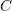, the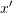axis is an axis crossing the centroid (a centroidal axis), and theaxis is an arbitrary axis parallel to.

If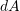is a differential element of the area, its (perpendicular) distance to theaxis can be written as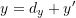where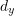is the distance between the two parallel axes shown in Fig. 10.9. Therefore,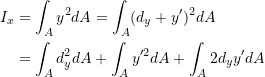The term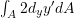equals zero because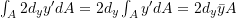and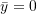(measured from theaxis) becausepasses through the centroid. Consequently,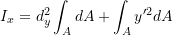With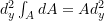and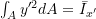, then

(10.7)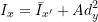which reads the moment of inertia about an axisis equal to the moment of inertia about a parallel axisthat crosses the centroid of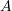, plus the product of areaand the square distance betweenand.

Equation 10.7 can be written for any two parallel axes with one crossing the centroid of the area. Ifis an axis crossing, anda parallel axis toas shown in Fig. 10.10a, we can write,

(10.8)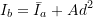where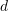is the distance between the two parallel axes.

The parallel axis theorem also hold for the polar moment of inertia. If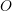is a point in the plane of an area and distantfrom the centroidof the area as shown in Fig. 10.10b, the polar moments of inertia aboutandare related as,

(10.9)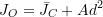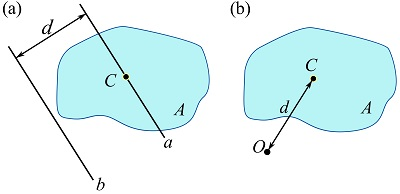Fig. 10.10 The parallel axis theorem for (a) the rectangular and (b) polar moments of inertia.

#### EXAMPLE 10.3.1

Determine the moment of inertia of a rectangular area about the x and y axes shown. Use the moment of inertia about the centroidal axes parallel to its sides.

#### SOLUTION

Letand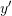be two axes crossing the centroid of the area as shown. According to Example 10.1.1,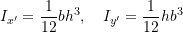Therefore, by Eq. 10.8, we can write,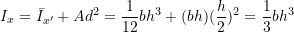And,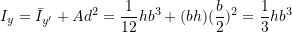#### EXAMPLE 10.3.2

If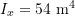for the depicted triangular area, determine the moment of inertia about the axis crossing the centroid of the area and parallel to the x axis.

#### SOLUTION

Find the distance from the x axis to the centroid of the area, and use Eq. 10.8.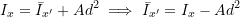Therefore,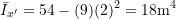Figure 10.11 demonstrates some basic areas and the formulations of their moments of inertia (see this document for some other areas).